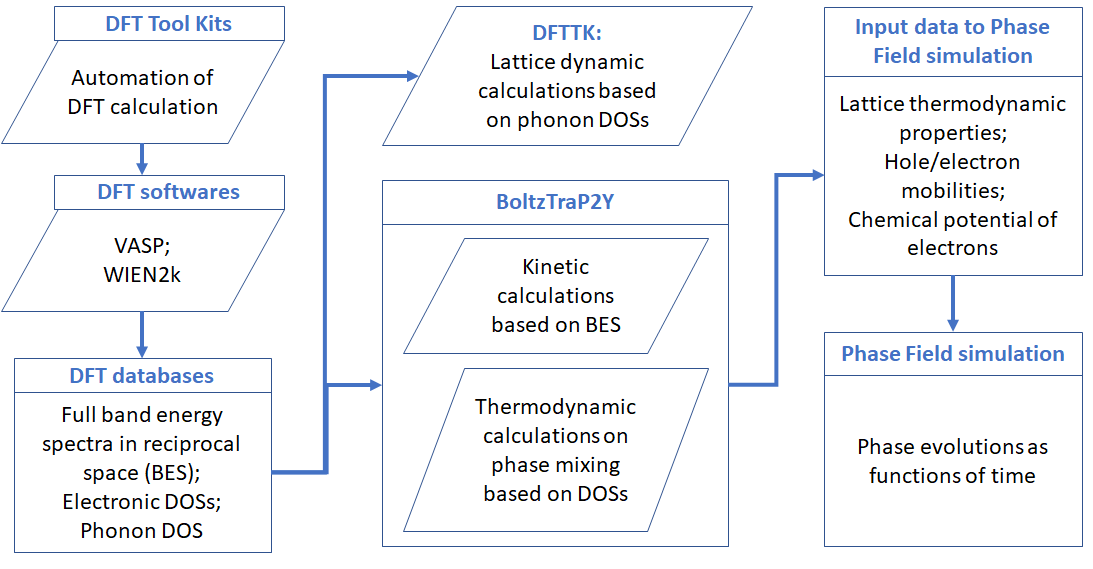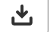The long term goal of this package is to bridge the data between DFT results, either in its original format or those save in MongoDB database, and the other simulations, for example, the phase-field simualtion of phase transition due to electronic structure change, typically, the metal insulator transition (MIT).The above figure shows a schematic demonstration of the technical procedure for the data flow of the package. This package was mainly implemented through the combination of several distinct approaches for accounting the roles of electrons and lattice vibrations:

1. the kinetic properties by means of solving the Boltzmann transport equation. The code was mainly based on forking the BoltzTraP2 by @georg.madsen et al. The major changes include the separation of the electron and hole contributions, in addition to technical improvements on the calculations of the chemical potential of electrons which makes it more efficient for calculation at low temperature that requires extremely refined meshes on electron energy.

2. the thermodynamic properties of electrons involving the mixing between two phases per our recent observations that certain eletron transfer related quantities, including:

which were thought to be dependent on the scattering mechanism and therefore can only be calcullated through the kinetic manner such as the Boltzmann transport equation, are in fact thermodynamic parameters and therefore can be predicted solely based on the electron density of states.

1. Our development for a package for the automation of first-principles calculations of thermodynamic properties of materials at finite temperature employing the quasi-hamonic phonon approach, as given in a separate documentaton (www.dfttk.org)

For more details, see the secction Penn State’s work.

## Quick run instruction¶

1. perform DFT calculations for, including a. 0-K electron energetics. These data contain the lattice structure, 0-K total energy, electronic density of states, electron energies in each k-mesh. b. interatomic force constants through supercell or linear response approach. These data will be used to calculate the lattice contribution to the thermodynamic properties.

2. invoke the BoltzTrap2Y package to calculate the kinetic/thermodynamic properties based on 0-K DFT results

3. when needed, invoke DFTTK to postprocess the interatomic force constants to get lattice contribution to the thermodynamic properties.

4. convert the data calculated by BoltzTrap2Y/DFTTK into the input to phase field simulation

## Run using Jupyter Notebook¶

To make ease of the package, we have prepared an extensive jupyter notebook script (which assumes that the step of DFT calculations have been completed) which can be excuted by the following steps

1. clicking the link BoltzTrap2Y.ipynb followed by click theicon in the right hand side of the web page. By default, a file named like `BoltzTrap2Y.ipynb` will be saved in your `Downloands` folder in the case of Windows computer. After that, go back to this page and run the codes using the free google notebook server by

2. clicking the link jupyter notebook google followed by uploading the downloaded code through clicking `file->upload` in the jupyter notebook google page.

3. clicking `Runtime->Run all` in the jupyter notebook google page

For more details, see the secction Example by Jupyter Notebook.

## Change logs in coding¶

As a numerical demonstration, we have implimented the computational procedure by python code through forking the BoltzTraP2 by @georg.madsen et al. The major extensions, which at present are only made for constant doping calculations, are briefed below:

1. revised `interface.py`

2. extended `bandlib.py` into `bandlibEXT.py`.

3. extended `io.py` into `ioEXT.py`

4. added `remesh.py` for calculations in low temperature (meshed increased to ~100,000 so that the calculated results can be good until a few of 10 K).

5. improved the computational efficiency on the calculations of the chemical potential of electrons (Fermi levels)

6. added `plotpng.py` for figure plot.